# Guess a fraction

Tom was asked to guess a fraction. The sum of 1/2 the numerator and 1/3 of its denominator is 30. If Tom subtracts 36 from its denominator, the fraction becomes 1/3. What is the fraction that Tom was asked to guess? (Leave your answer in simplest form)

d =  72
n =  12
f =  0.1667

### Step-by-step explanation:

n/2+d/3=30
n= (d-36)/3

2d+3n = 180
d-3n = 36

d = 72
n = 12

Our linear equations calculator calculates it.
$f=n\mathrm{/}d=12\mathrm{/}72=\frac{1}{6}=0.1667$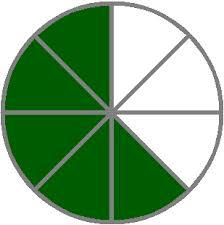Did you find an error or inaccuracy? Feel free to write us. Thank you!

Showing 1 comment:Math student
can say in a simpler form

1 year ago  1 LikeTips to related online calculators
Need help to calculate sum, simplify or multiply fractions? Try our fraction calculator.
Do you have a linear equation or system of equations and looking for its solution? Or do you have a quadratic equation?

## Related math problems and questions:

• Simplest form of a fractionWhich one of the following fraction after reducing in simplest form is not equal to 3/2? a) 15/20 b) 12/8 c) 27/18 d) 6/4
• Division by zero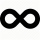Fraction 5 by 2. if 3 is added to numerator and 2 is subtracted from the denominator then the new fraction is:
• Simplest form 3What fraction is 15 of 35 in simplest form?
• Equivalent fractions 2Write the equivalent multiplication expression. 2 1/6÷3/4
• Fractions and mixed numerals(a) Convert the following mixed numbers to improper fractions. i. 3 5/8 ii. 7 7/6 (b) Convert the following improper fraction to a mixed number. i. 13/4 ii. 78/5 (c) Simplify these fractions to their lowest terms. i. 36/42 ii. 27/45 2. evaluate the follow
• Denominator 2Denominator of a fraction is 5 and numerator is 7. Write the fraction .
• Reducing scale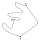I am sizing some landscaping and I measured everything for a 20 to 1 scale. I realized when I was done that it was at 30 to 1. How can I convert what I already have to 30 to 1. (Example, grass area is 22,871.6' at 20 to 1, how do you figure it out to be 3
• What fraction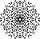What fraction of number 1 to 30 is prime?
• Evaluate 17Evaluate 2x+6y when x=- 4/5 and y=1/3. Write your answer as a fraction or mixed number in simplest form.
• Two simple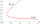Two simple fraction have the product of 3/10. When the smaller fraction is divided by the bigger fraction, the quotient is 5/6. What are the two fractions in simplest form?
• Find unknown 2Find unknown denominator: 2/3 -5/? = 1/4
• Fraction of a NumberIf 1/2 of 1/3 of 1/4 of 1/5 of a number is 2.5. what is the number?
• When 5When 5 is subtracted from both my numerator and denominator I become 3/4. What fraction i am?
• 30 eggs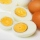There are 30 eggs in a tray. If 1/2 of the tray used 1/5 of it cooked,1/3 kept the refrigerator, how many eggs were left?
• Nick was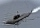Nick was scuba diving at -32 1/2 feet. If he descends another 8 3/5 feet, what is his location?
• Evaluate mixed expressionsWhich of the following is equal to 4 and 2 over 3 divided by 3 and 1 over 2? A. 4 and 2 over 3 times 3 and 2 over 1 B. 14 over 3 times 2 over 7 C. 14 over 3 times 7 over 2 D. 42 over 3 times 2 over 31
• A turtle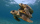A turtle is 20 5/6 inches below the surface of a pond. It dives to a depth of 32 1/4 inches. What is the change in the turtle’s position? Write your answer as a mixed number.